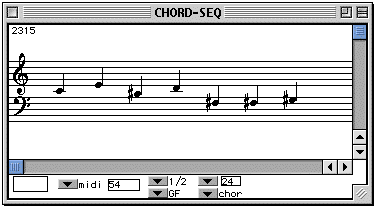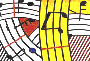Tutorial 7 - Expansion and compression of intervals in a sequence

Topics

Scaling intervals of a sequence of notes by reducing or expanding each interval using arithmetical and serial operators.

Functions used

CHORD
, CHORD-SEQ, dx->x, om*, first, om-round, x->dx.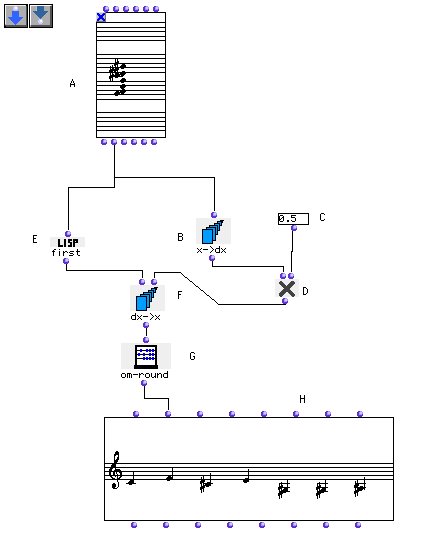Description

This patch makes either an expansion or a compression of the intervals in a series of notes. The box x->dx (B) calculates the intervals of the sequence of notes (A). Then om* (D) changes these intervals proportionally by multiplying them by a constant in the t box (C). The result of the multiplication is a new list of intervals that, once entered in the dx->x box (F) can be used to create a new series of notes.

Patch structure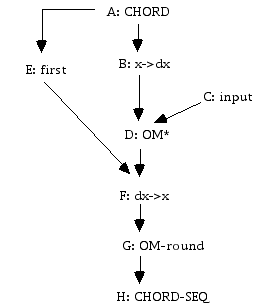A: Open the CHORD box by clicking in the centre of the box and edit a sequence of notes in the "order" mode. Then lock it.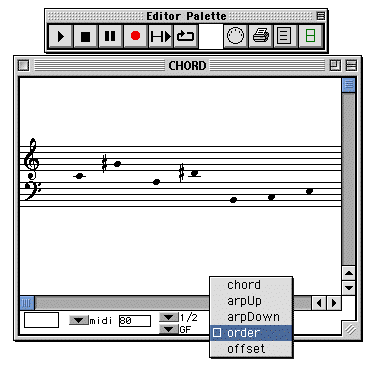B: The x->dx box calculates the intervals between the notes in the sequence (A). The x->dx box calculates the differences between each pair of notes in the scale defined in (A). If you have the sequence DO-RE-MI-FA-SOL, the difference between DO and RE will be 200 (two semitones), between RE and MI, 200 (again, two semitones), and between MI and FA, 100 (one semitone) By doing this you calculate the intervals between the notes of your sequence.

C: Enter a multiplication factor for the expansion or compression of the intervals in this input. If you enter a number between 0 and 1 (for example 0.2 0.365 0.99999 etc) the note series will be compressed. On the contrary, if you enter a number greater than 1 (for example 2, 2.00001, 10 etc) the series will be expanded. (A value of 1 will neither compress nor expand the series.)

D: The om* box multiplies the intervals coming from the x->dx box by the value entered in (C).

E: The first box (E) takes the first note of the CHORD box (A) (remember it will be the first note edited in a CHORD box, and not necesseraly the lowest one). This note will be the starting note value for the compressed or expanded series.

F: The dx->x module makes the new sequence, starting with the value coming from first box (E), and constructed using the intervals deriving from the x->dx box.

G: The om-round rounds the values coming out of the dx->x module.

H: Evaluate and open the CHORD-SEQ to see the result.